# Equation - 7th grade (12y) - math problems

#### Number of examples found: 516

• Line segmentCut a line segment of 15 cm into two line segments so that their lengths are in ratio 2:1. What length will each have?
• Blueberries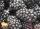Miko and Anton have a total of 1,580 blueberries. Miko and Anton have them in the ratio 2: 3. Determine how much each of them has.
• Football seasonThroughout the football season gave Adam the 23 goals more than Brano and 16 goals less than Karol. Edo gave the 6 goals more Dušan. Karol gave 5 more than Edo. Brano and Edo gave together 17 goals. How many goals gave each boy and how many goals gave the
• Translations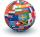If I going to translate the book 6 pages per day I translate it 4 days earlier than if I translated 5 pages a day. If I translate 4 pages a day I translate it for how many days.....?
• ExpressionIf it is true that ? is:
• My fatherMy father has a big farm. 6/8 of it were planted with mango trees, 1/2 of the remainder are guava trees and the rest 10 trees are santol trees. What is the number of all trees?
• Map scaleThe rectangular plot has in a scale of 1: 10000 area 3 cm2 on the map. What content does this plot have on a 1:5000 scale map?
• Complementary angles 2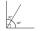Two complementary angles are (x+4) and (2x - 7) find the value of x
• Calf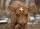The weight of the calf meat obtained is 65% of the total weight of live calves. What was the weight of a calf from which 285 kg of meat was obtained?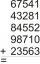Fill letters instead of digits so the indicated sum (equal letters represent equal digits). What number is hidden under the letter J? A A H A H O A H O J -------------------------- 4 3 2 1
• Unknown number 5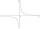Daniel think an integer. When he change this number at a ratio of 2:5 he got number 2.8. Determine what number think Daniel.
• Suit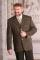The suit cost 119 euros. How much cost the pants if it is 50% cheaper than the jacket.
• Unknown number 4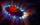I think number. When I divided its square root by its 1/9, I get a number 1. What number am I thinking?
• Bob and Bobek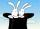At 10h 20min runs Bobek from hat at average speed 12 km/h. At what time must run out Bob at speed 18 km/h to catch him 9 kilometers from the hat?
• Grandmother and grandfatherGrandmother baked cakes. Grandfather ate half, then quarter of the rest ate Peter and Paul ate half of rest. For parents left 6 cakes. How many cakes maked the grandmother?
• Price increase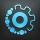A 20 percent price increase meant a 90-crown raise. How many cost a product after?
• Bar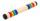Half of the bar is green, the third is yellow and the remaining 40cm is red. How long is a bar?
• Dividing moneyVilem, Cenek, and Edita divided the money they earned by spreading the leaflet. Vilem got 240 CZK more than Cenek and twice more than Edita. Edita got 400kc less than Vilem.
• Solve 2Solve integer equation: a +b+c =30 a, b, c = can be odd natural number from this set (1,3,5,7,9,11,13,15)
• Simple equation 324 = n • 27, solve for n

Do you have an interesting mathematical word problem that you can't solve it? Submit a math problem, and we can try to solve it.

We will send a solution to your e-mail address. Solved examples are also published here. Please enter the e-mail correctly and check whether you don't have a full mailbox.

Please do not submit problems from current active competitions such as Mathematical Olympiad, correspondence seminars etc...
Do you have a linear equation or system of equations and looking for its solution? Or do you have quadratic equation?